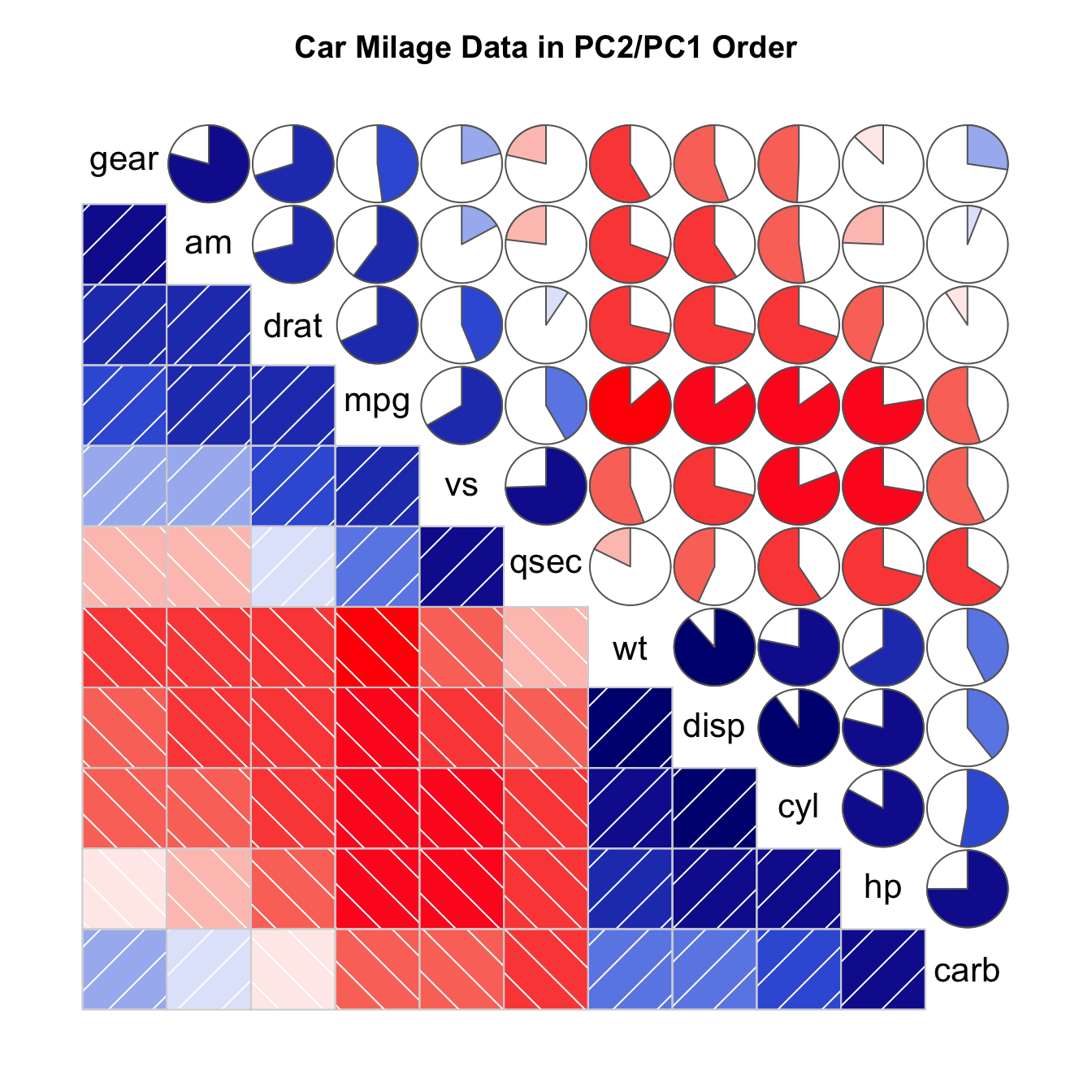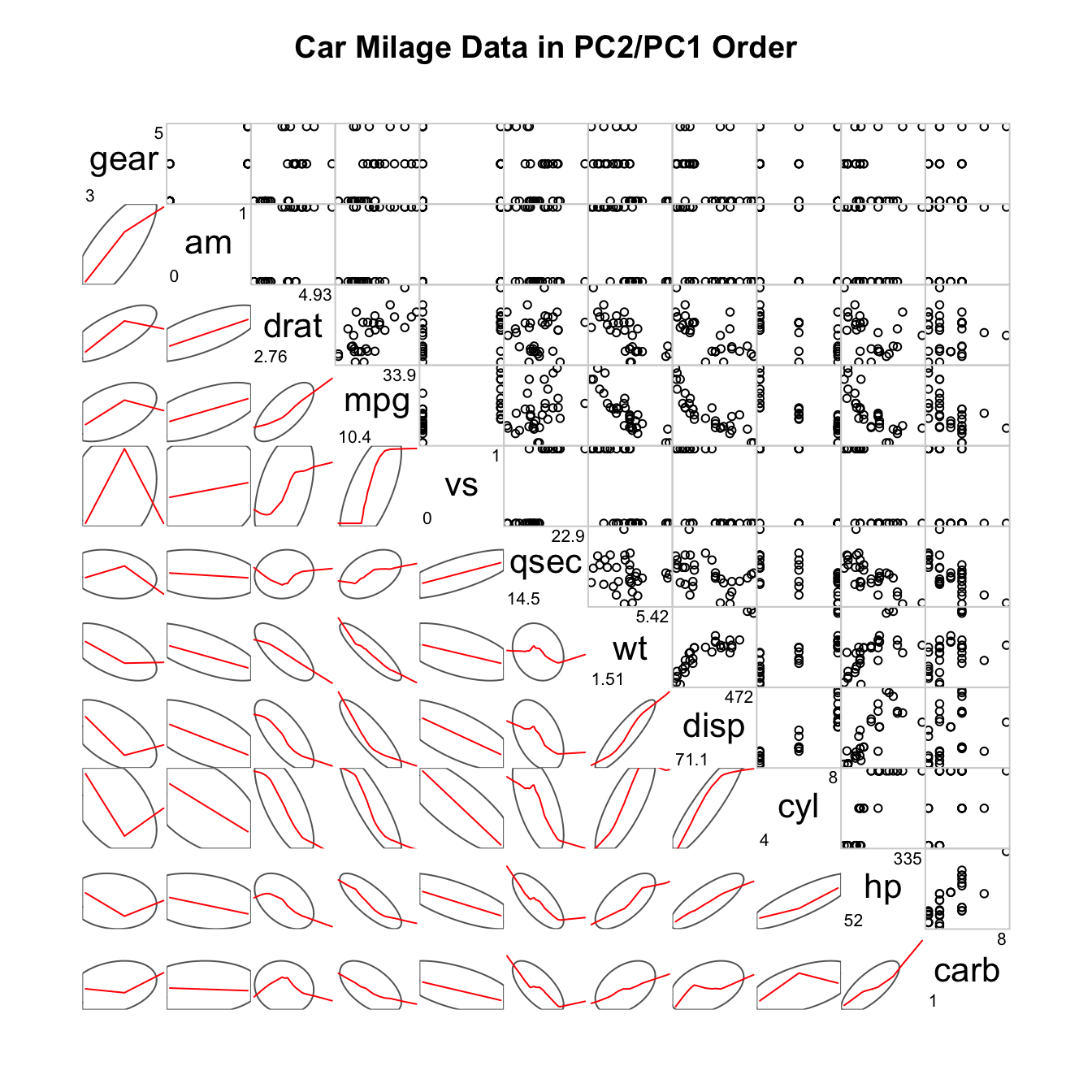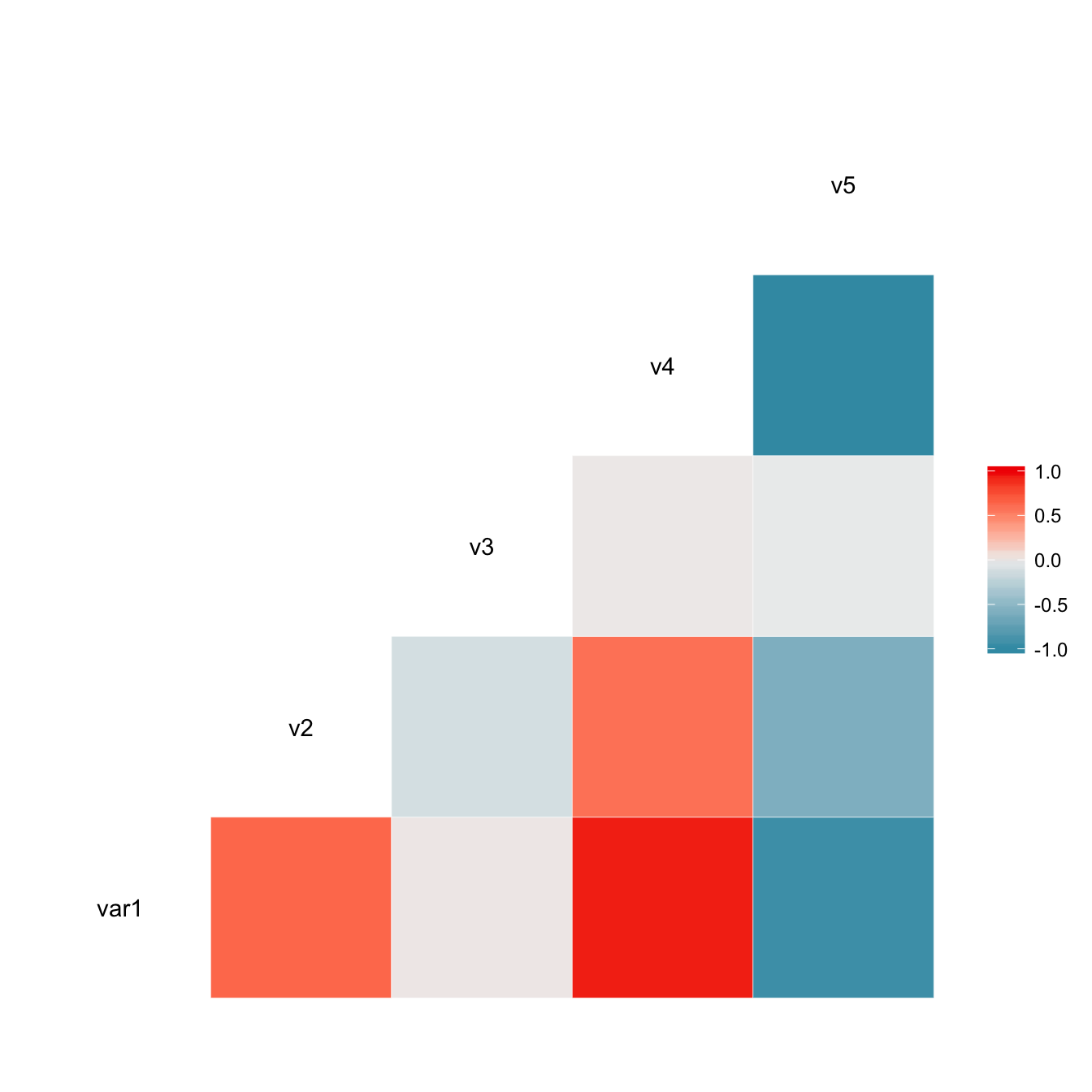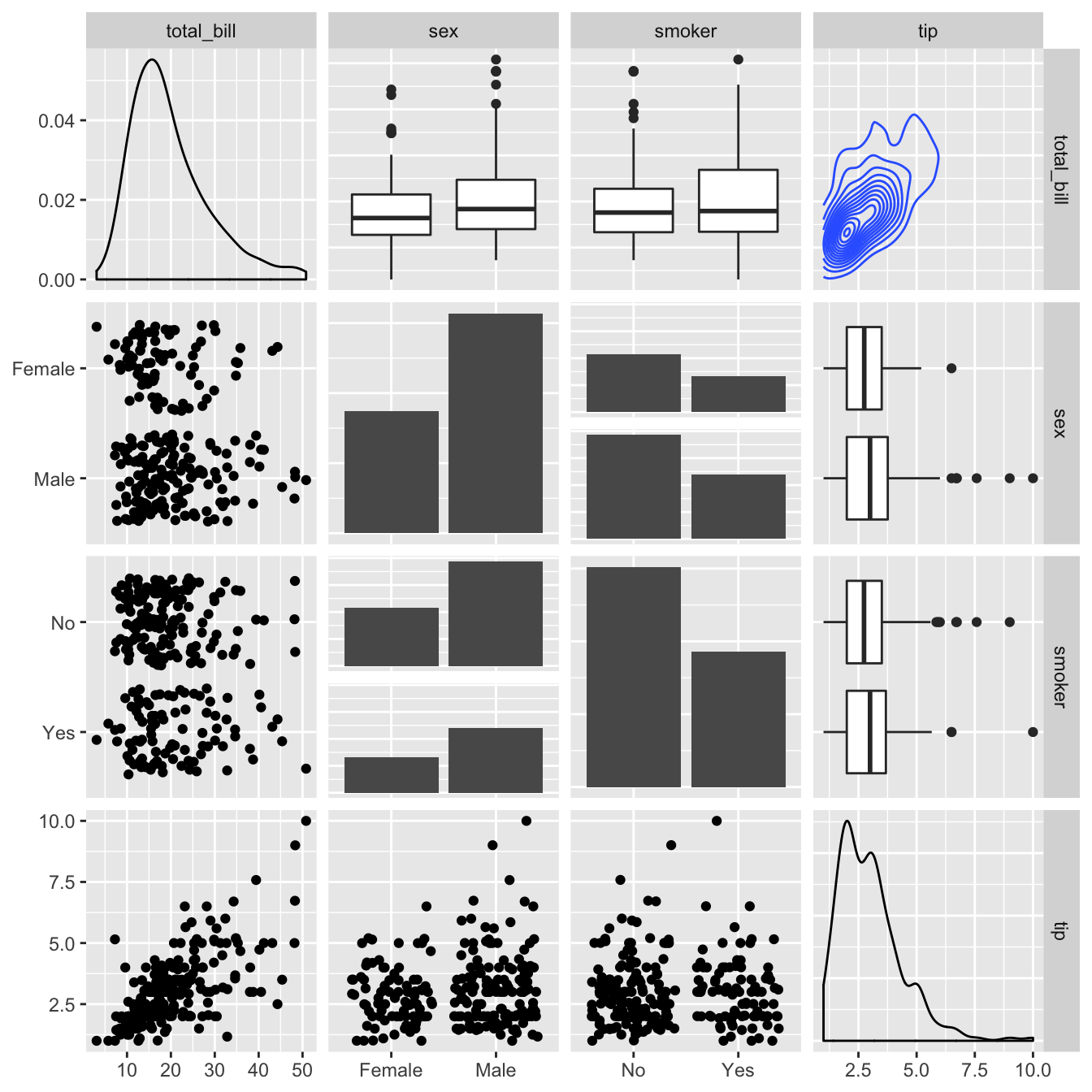# Correlogram with the corrgram library

This post explains how to build a correlogram with the `corrgram` R package. It provides several reproducible examples with explanation and `R` code.

# Scatterplot matrix with `ggpairs()`The `corrgram` package allows to build correlogram. The output allows to check the relationship between each pair of a set of numeric variable.

Relationship can be visualized with different methods:

• `panel.ellipse` to display ellipses
• `panel.shade` for coloured squares
• `panel.pie` for pie charts
• `panel.pts` for scatterplots
``````# Corrgram library
library(corrgram)

# mtcars dataset is natively available in R

# First
corrgram(mtcars, order=TRUE, lower.panel=panel.shade, upper.panel=panel.pie, text.panel=panel.txt, main="Car Milage Data in PC2/PC1 Order")

# Second
corrgram(mtcars, order=TRUE, lower.panel=panel.ellipse, upper.panel=panel.pts, text.panel=panel.txt, diag.panel=panel.minmax, main="Car Milage Data in PC2/PC1 Order")

# Third
corrgram(mtcars, order=NULL, lower.panel=panel.shade, upper.panel=NULL, text.panel=panel.txt, main="Car Milage Data (unsorted)")``````

# Visualize correlation with `ggcorr()`

The `ggcorr()` function allows to visualize the correlation of each pair of variable as a square. Note that the `method` argument allows to pick the correlation type you desire.``````# Quick display of two cabapilities of GGally, to assess the distribution and correlation of variables
library(GGally)

# Create data
data <- data.frame( var1 = 1:100 + rnorm(100,sd=20), v2 = 1:100 + rnorm(100,sd=27), v3 = rep(1, 100) + rnorm(100, sd = 1))
data\$v4 = data\$var1 ** 2
data\$v5 = -(data\$var1 ** 2)

# Check correlation between variables
#cor(data)

# Nice visualization of correlations
ggcorr(data, method = c("everything", "pearson"))``````

# Split by group

It is possible to use ggplot2 aesthetics on the chart, for instance to color each category.``````# Quick display of two cabapilities of GGally, to assess the distribution and correlation of variables
library(GGally)

# From the help page:
data(flea)
ggpairs(flea, columns = 2:4, ggplot2::aes(colour=species))``````

# Change plot types

Change the type of plot used on each part of the correlogram. This is done with the `upper` and `lower` argument.``````# Quick display of two cabapilities of GGally, to assess the distribution and correlation of variables
library(GGally)

# From the help page:
data(tips, package = "reshape")
ggpairs(
tips[, c(1, 3, 4, 2)],
upper = list(continuous = "density", combo = "box_no_facet"),
lower = list(continuous = "points", combo = "dot_no_facet")
)``````

Related chart types

## Contact

This document is a work by Yan Holtz. Any feedback is highly encouraged. You can fill an issue on Github, drop me a message on Twitter, or send an email pasting yan.holtz.data with gmail.com.